# Intro to Neural Networks

## Introduction

Artificial neural networks are one of the most powerful tools used in machine learning and artificial intelligence. Inspired by biological neural networks, they can tackle a wide range of problems that defeat traditional AI, including computer vision, speech recognition, natural language processing, and robotics.

In general, neural networks can be used for any task involving pattern recognition, classification, and prediction. They are being deployed on a large scale by companies like Google, Microsoft, and Facebook. They also underlie cutting-edge projects like AlphaGo, a project of Google DeepMind, whose deep neural network was the first to beat a human professional Go player.

In this post, we will help you master the core concepts behind neural networks: how they work, how to train them, and how to apply them to problems.

## What is a neural network?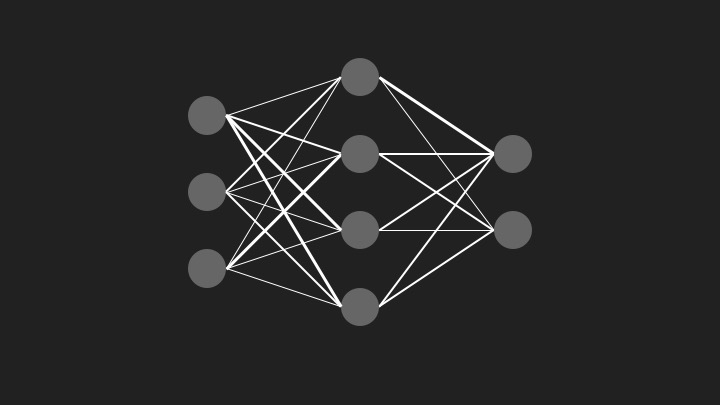A neural network is a system of interconnected neurons that can send signals to each other. The strengths of the connections between these neurons, also known as weights, determine how the network behaves. Most importantly, these weights can be systematically tuned to make the neural network behave in a highly specific, desirable way.

For example, consider the problem of making a program that can recognize handwritten digits. A neural network for handwriting recognition could consist of three separate layers of neurons: an input layer, a hidden layer, and an output layer. Each layer of neurons is fully connected to the next.

In our problem, the input layer would be a set of neurons which represent each pixel of the input image. It is the ‘eye’ through which the neural network sees an image, similar to the way a human retina is made of multiple photoreceptor cells. The neurons in the input layer are excited (or fired or activated), according to the color of the corresponding pixel. A white pixel, for example, could cause the corresponding input neuron to activate fully.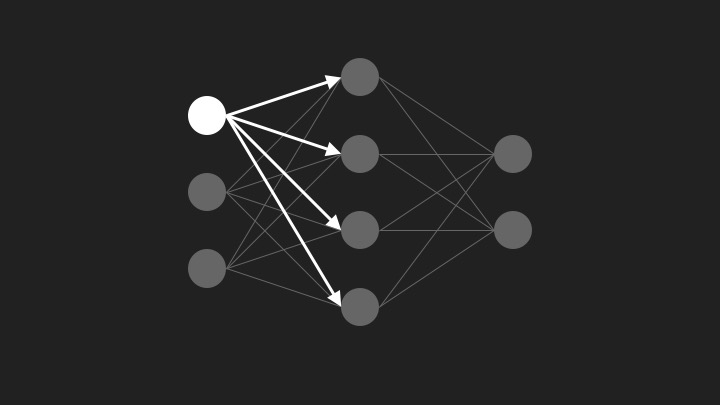Each activated neuron in the input layer sends a signal to all the neurons in the next layer, which is the hidden layer. The strength of the signal is dependent on both how much the neuron is activated and on the weight of the connection between the two neurons (one in the input layer and one in the hidden layer).

Each neuron in the hidden layer then adds up all of the signals it received from neurons in the input layer. It then applies something called a transfer function or activation function to this sum. Examples of activation functions include sigmoids (which ‘squish’ the input into some range) like the logistic function and the hyperbolic tangent function, as well as the step function. This activation function introduces non-linearity into the network and allows it to represent functions which are non-linear. Symmetric sigmoids such as hyperbolic tangent often converge faster than the standard logistic function (see section 4.4 in this paper).

After each neuron in the hidden layer applies an activation function to its input, it in turn sends a signal to all the neurons in the next layer (the output layer). Each neuron in the output layer corresponds to a different possible digit (i.e. 0, 1, 2, …, 8, 9). The output neuron which has the highest activation will represent which digit the neural network thinks the image contains.

## Bias neurons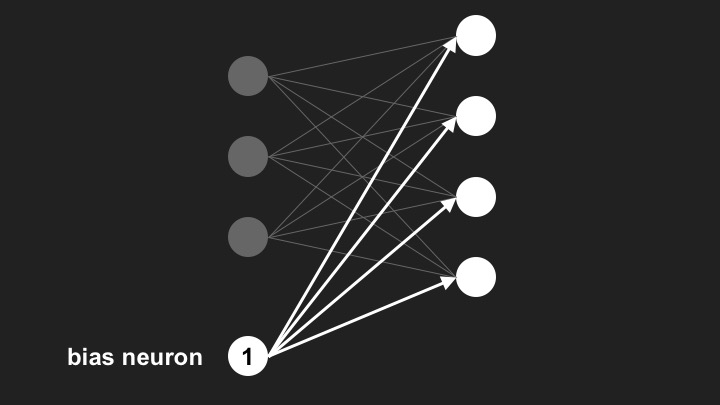Bias neurons allow the network output to be translated to the desired output range. A bias neuron is simply a neuron with an activation set to the maximum possible value. This value, along with those of all the other neurons in the same layer, is passed along to all neurons in the next layer. The function of the bias neuron is to shift the input sums in the next layer by a value that is independent of the input to the neural network. This allows the network to represent functions which are affine in nature. Essentially, its effect is the same as shifting the activation functions of the neurons in the next layer horizontally. This allows us to translate the output to the desired output range.

An elegant way to incorporate bias neurons into our code is to represent them as vectors which are added to the input vector of the neurons in the next layer. This is because the bias neurons are simply adding a fixed value to each neuron in the following layer.

## Training a neural network

Neural networks are “trained” by adjusting the strengths of the connections, or weights, between neurons. Backpropagation is a particularly useful and elegant algorithm for doing this. It is a special case of the more general concept of gradient descent. The idea behind gradient descent is to find the minimum of some function by slowly “descending” in the direction opposite to the gradient. You can think of it as a hiker making their way down a mountain by following the slope in the direction of steepest descent.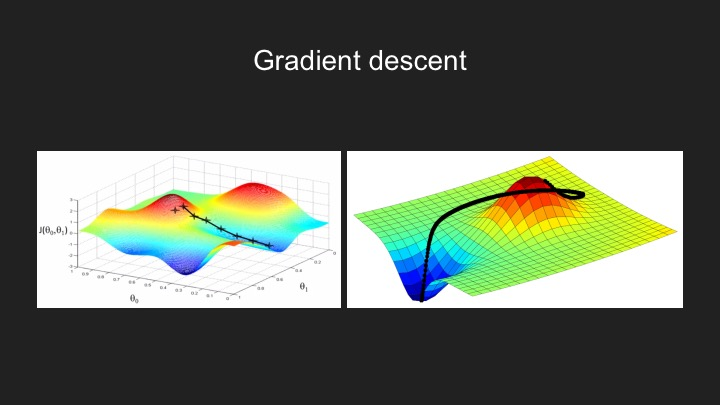On a high-level view, a neural network “learns” more or less the same way a child learns when presented with a new concept. A teacher, or supervisor, presents the learner with a series of samples, asking the learner to classify them. Initially, the learner performs quite poorly and classifies the samples more or less randomly. However, as the learner encounters more and more examples, each time comparing their guess to the answer, it becomes better and better at identifying the correct answer.

Backpropagation is simply a way of minimizing the loss function, or error, of the network by propagating errors backward through the network and adjusting weights accordingly. First, the difference between the actual output and the expected or desired output at the last layer is calculated. The algorithm then works backward through the network, calculating how changing a weight would change the overall error at the output layer.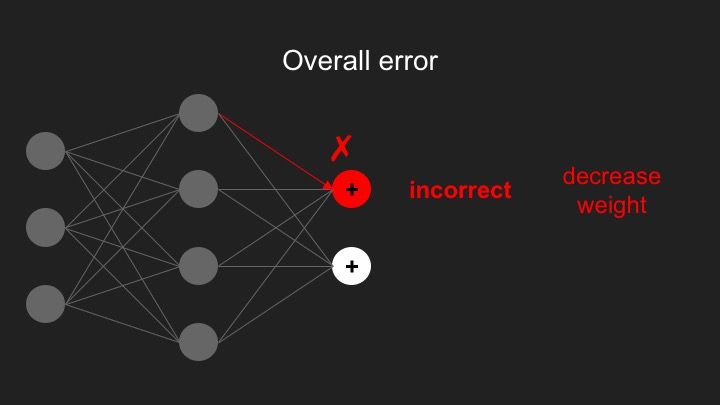For example, if a particular weight or connection in the network was contributing greatly to a large error, its strength would be reduced by the algorithm. In the next iteration, the neural network’s output should be closer to the desired output.

The dataset that specifies the desired inputs and outputs is called the training data or training set. Algorithms such as backpropagation are iterative, meaning that they converge to a better weight configuration each time they are run on the data set.

## Overfitting and cross-validation

Overfitting occurs when the neural network continues to learn its training set almost perfectly but starts performing worse on unseen data. The reason this occurs is because the neural network starts learning the noise or error in the data rather than the underlying relationship. This usually occurs when the neural network learns a model that has too many parameters relative to the size of the data.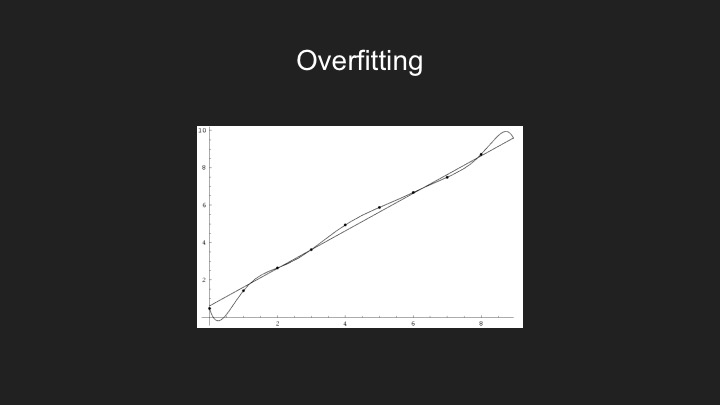An example of overfitting can occur when trying to fit a polynomial to a set of data points. For example, consider a linear fit that has relatively small error and, although it fails to pass through all of the data points, generalizes the underlying trend quite well. On the other hand, one could use a high-degree polynomial and force it to go through every data point. However, it is likely that this high-degree polynomial would be worse at generalizing and extrapolating the data, compared to the linear fit.

Overfitting, or overtraining, occurs similarly in neural networks. It can be prevented by using a technique called cross-validation. The idea behind cross-validation is to split the training set into two: a set of examples to train with, and a validation set to test against. The neural network trains using the training set, whereas it is tested on the validation set. Prediction accuracy on the validation set is used to determine which model to use.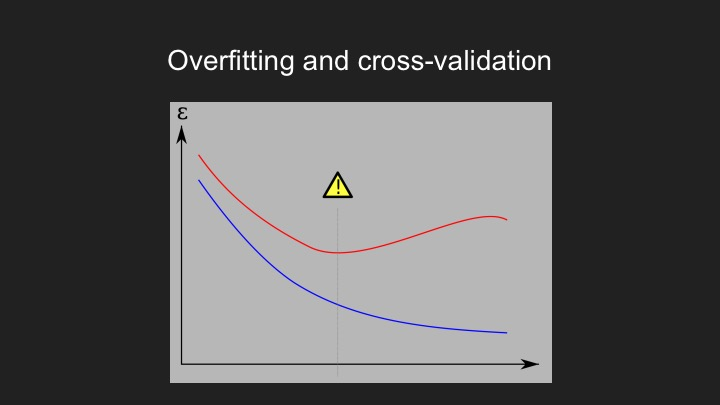Overfitting occurs when the performance of the network continues to improve on the training set, while its performance on the validation (or testing) set begins to deteriorate. In other words, the error decreases on training data, while it begins to increase on the validation data. The training of the neural network should stop once the validation error has reached its global minimum, to prevent overtraining.

## How many hidden neurons should I use?

There is no universal shortcut for determining what is the best number of neurons in the hidden layer to use. A good number tends to be somewhere between the number of input neurons and the number of output neurons (such as a mean of those two). The best way to figure out the ‘optimal’ number of neurons to use would be to test how different neural network structures (with different numbers of neurons in the hidden layer) perform on your particular problem, using the concept of cross-validation: Train your neural network on a subset of your total data, and then test it on a different subset of your data that it has not seen before.

There are programmatic ways to ‘prune’ your neural network by starting off with a large number of hidden neurons and then reducing this number until the performance of the network begins to deteriorate significantly (or falls below some threshold of accuracy). Empirical testing would be the best way to approach this problem.

## Python example

We have created a small python program which trains a simple feedforward neural network by means of backpropagation to recognize images of handwritten numbers. This code has no external dependencies beyond numpy (a Python library for numerical computation) and matplotlib (a Python library for plotting), and is meant to demonstrate the simplicity of implementing a basic feedforward neural network. The code is available on Github here.

— Carlos Martin, Lucas Schuermann
Based upon material from our "Accessible Machine Learning: Neural Networks" lecture given at Columbia University, 2/24/16.

Last updated May 16, 2020